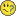# New to Qlik Sense

Discussion board where members can get started with Qlik Sense.

Announcements
Make your voice heard! Participate in the 2020 Wisdom of Crowds® Survey. BEGIN SURVEY
Highlighted
New Contributor III

## if condition set analysis

Hi all,

I am trying to do something like

if(var=0, count({<[V.Month]={'\$(=Max([V.Month]))'}>} distinct ItemID),

if(var=1, count({\$<"Found in X field"={'Yes'}>*<[V.Month]={'\$(=Max([V.Month]))'}>} distinct ItemID),

if(var=2, count({\$<"Found in Y field"={'Yes'}>*<[V.Month]={'\$(=Max([V.Month]))'}>} distinct ItemID))))

for a bar chart which shows the latest month data. I only want to include the 'Yes' values in the "Found in X field" for this bar chart, hence the if statement.

Without the if condition the below expression works

count({\$<"Found in X field"={'Yes'}>*<[V.Month]={'\$(=Max([V.Month]))'}>} distinct ItemID)

Any suggestions on how to approach this? Thank youTags (3)
1 Solution

Accepted Solutions
Highlighted
New Contributor III

## Re: if condition set analysis

Found this document helpful and managed to (understand set analysis better) display the correct numbers!https://community.qlik.com/docs/DOC-4951

Used the expression belowCount({\$<[V.Month]={'\$(=Max([V.Month]))'}, varMonth={"0"} >+<[V.Month]={'\$(=Max([V.Month]))'}, "Found in 2nd mth?"={'Yes'}, varMonth={"1"}>+<[V.Month]={'\$(=Max([V.Month]))'}, "Found in 3rd mth?"={'Yes'}, varMonth={"2"} >} distinct [%ItemID])

Thanks all for the help!

8 Replies
Highlighted
Esteemed Contributor

## Re: if condition set analysis

Hi,

can you add sample data ? var is a variable ?

Highlighted
New Contributor III

## Re: if condition set analysis

Hi,

Am afraid it would be too tedious to upload a sample file as I have confidential data and a few mapping tablesvar is a calculated field of either 0,1 or 2. The Found in X/Y fields are calculated fields as well and are either 'Yes' or 'No'.

HighlightedMVP

## Re: if condition set analysis

If you "only want to include the 'Yes' values in the "Found in X field" for this bar chart, hence the if statement." and the single aggregation

count({\$<"Found in X field"={'Yes'}>*<[V.Month]={'\$(=Max([V.Month]))'}>} distinct ItemID)

returns the correct result ("it works"), what's your exact issue? Why do you need the if() Statement at all?

Highlighted
New Contributor III

## Re: if condition set analysis

Oops my bad, I meant I only want to include the 'Yes' values in the "Found in X field" as well as for the "Found in Y field" for this bar chart.

I need the if statement as the set analysis depends on the var field.

Eg. var=0 => No need to filter out "Found in __ field"

var=1 => Need to filter out "No" values from "Found in X field"

var=2 => Need to filter out "No" values from "Found in Y field"

HighlightedMVP

## Re: if condition set analysis

If you are saying, that var is a calculated field, you mean that you need to consider this field (check against its value) on a per record base?

As Youssef already mentioned, some more details, like some sample data (we don't need you full and real data, but anything that explains your Setting), would really help us to help you.

New Contributor III

## Re: if condition set analysis

Hi Stefan,

Yes I believe that is what I am trying to achieve.

The bar chart's Dimensions are "Business Domain" and Rating (High/Medium/Low). I am trying to get the Measure to calculate the total values for only 'Yes' values unless var=0.

var, Found in X and Y are derived as follows:

if(SubField(Round(Num(Month(\$(vMonth)))/3, 0.01),'.',2)=33, 0,

if(SubField(Round(Num(Month(\$(vMonth)))/3, 0.01),'.',2)=67, 1,

2)) as varMonth // \$(vMonth) is taken from the file name

if(varMonth=0 or "First Discovered"=Null(), Null(), /if 1st month or First Discovered field is null then null

if(isNull(ApplyMap('mapTrue1stDate', VulnIDMth, Null())), 'No', //if 2nd/3rd month and ApplyMap is null => no

'Yes')) as "Found in X",

if(varMonth=0 or varMonth=1 or "First Discovered"=Null(), Null(), //if 1st or 2nd month then null

if(isNull(ApplyMap('mapTrue1stDate', VulnIDMth, Null())), 'No',  //if 3rd month and ApplyMap is null => no

'Yes')) as "Found in Y",

Hope the mentioned details are relevant. Thanks!

Highlighted
New Contributor III

## Re: if condition set analysis

Found this document helpful and managed to (understand set analysis better) display the correct numbers!https://community.qlik.com/docs/DOC-4951

Used the expression belowCount({\$<[V.Month]={'\$(=Max([V.Month]))'}, varMonth={"0"} >+<[V.Month]={'\$(=Max([V.Month]))'}, "Found in 2nd mth?"={'Yes'}, varMonth={"1"}>+<[V.Month]={'\$(=Max([V.Month]))'}, "Found in 3rd mth?"={'Yes'}, varMonth={"2"} >} distinct [%ItemID])

Thanks all for the help!

HighlightedPartner

## Re: if condition set analysis

Hi,

Can anyone help me out for the below expression to write using set analysis.